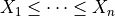# Create a maximum entropy statistics distribution¶

In this example we are going to build maximum entropy statistics distribution, which yields ordered realizations:import openturns as ot
import openturns.viewer as viewer
from matplotlib import pylab as plt

ot.Log.Show(ot.Log.NONE)


create a collection of distribution

coll = [ot.Beta(1.5, 1.7, 0.0, 1.0), ot.Beta(2.0, 2.3, 0.5, 1.2)]


create the distribution

distribution = ot.MaximumEntropyOrderStatisticsDistribution(coll)
print(distribution)

MaximumEntropyOrderStatisticsDistribution(collection = [Beta(alpha = 1.5, beta = 1.7, a = 0, b = 1),Beta(alpha = 2, beta = 2.3, a = 0.5, b = 1.2)])


draw a sample (ordered!)

distribution.getSample(10)

X0 X1 0.6380705 0.7940242 0.3118801 0.8304515 0.4525162 0.6586167 0.6724817 0.8490304 0.2977258 0.9140666 0.1691129 0.9547993 0.3545962 0.9400742 0.8338034 0.8877587 0.1830482 0.8514765 0.5859367 0.8921155

draw PDF

graph = distribution.drawPDF()
view = viewer.View(graph)
plt.show()